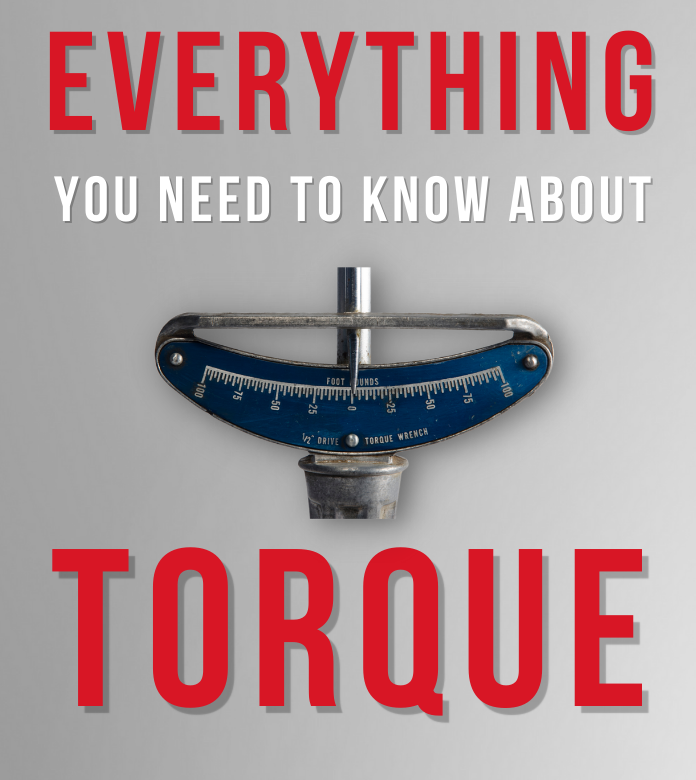# Torque Conversion CalculatorWhat is torque? Torque is a form of measurement used for rotational or turning force, as in the amount of force it takes to cause an object to rotate around an axis. How many inch-pounds are in 1 foot-pound of torque? There are 12 inch-pounds in 1 foot-pound. To convert 1 foot-pound to inch-pounds multiply your number by 12. How to convert inch-pounds to foot-pounds? One foot-pound is equal to 12 inch-pounds. To convert your torque measurements of inch-pounds to foot-pounds, divide your number by 12. To convert from foot-pounds to inch-pounds, just multiply by 12. How to convert a newton meter to foot-pounds? The conversion rate between newton meter and foot-pound is a combination of the ratios of feet to meters and pounds to newtons. The conversion rate is 1 newton meter equals 0.738 foot-pounds and 1 foot-pound equals 1.356 newton meters.To learn more about Torque and how it relates to industrial assembly tools